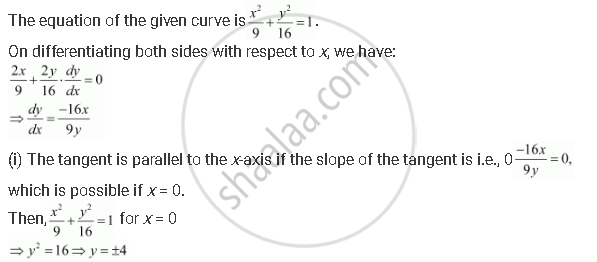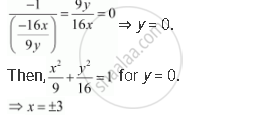Share

# Find Points on the Curve X^2/9 + Y^2/6 = 1 at Which the Tangents Are - CBSE (Commerce) Class 12 - Mathematics

#### Question

Find points on the curve x^2/9 + y^2/6 = 1 at which the tangents are

(i) parallel to x-axis

(ii) parallel to y-axis

#### SolutionHence, the points at which the tangents are parallel to the x-axis are (0, 4) and (0, − 4).

(ii) The tangent is parallel to the y-axis if the slope of the normal is 0, which givesHence, the points at which the tangents are parallel to the y-axis are (3, 0) and (− 3, 0).

Is there an error in this question or solution?

#### APPEARS IN

NCERT Solution for Mathematics Textbook for Class 12 (2018 to Current)
Chapter 6: Application of Derivatives
Q: 13 | Page no. 212

#### Video TutorialsVIEW ALL 

Solution Find Points on the Curve X^2/9 + Y^2/6 = 1 at Which the Tangents Are Concept: Tangents and Normals.
S